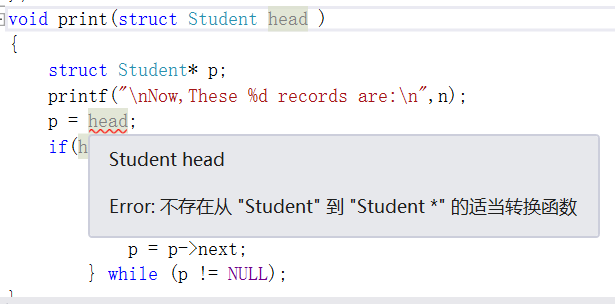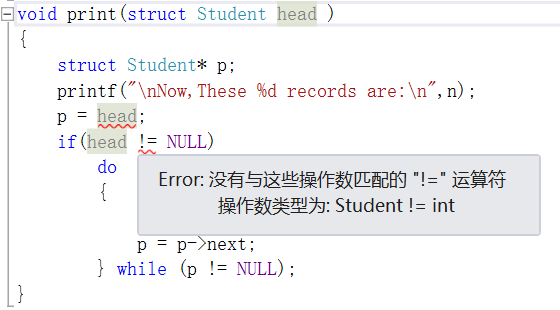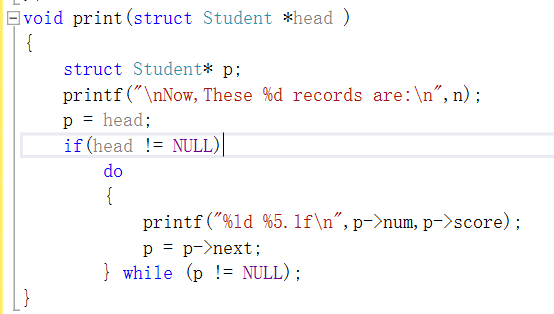# VS2012 Error:不存在从“——”到“——*”的适当转换函数—已解决

#include<stdio.h>
#include<malloc.h>
#define LEN sizeof(struct Student)
struct Student
{
long num;
float score;
struct Student* next;
};
int n;
struct Student* creat()
{
struct Student* p1,*p2;
n = 0;
p1 = p2 =(struct Student*)malloc(LEN);
scanf_s("%ld,%f",&p1->num,&p1->score);
while (p1 -> num!= 0)
{
n = n + 1;
else p2 -> next = p1;
p2 = p1;
p1 = (struct Student*)malloc(LEN);
scanf_s("%ld,%f",&p1 -> num,&p1 -> score);
}
p2 -> next = NULL;
};
{
struct Student* p;
printf("\nNow,These %d records are:\n",n);
do
{
printf("%ld %5.1f\n",p->num,p->score);
p = p->next;
} while (p != NULL);
}
void main()
{
}©️2019 CSDN 皮肤主题: 大白 设计师: CSDN官方博客